# Balancing Equations Chemical Reactions l Chemical rxns occur

• Slides: 17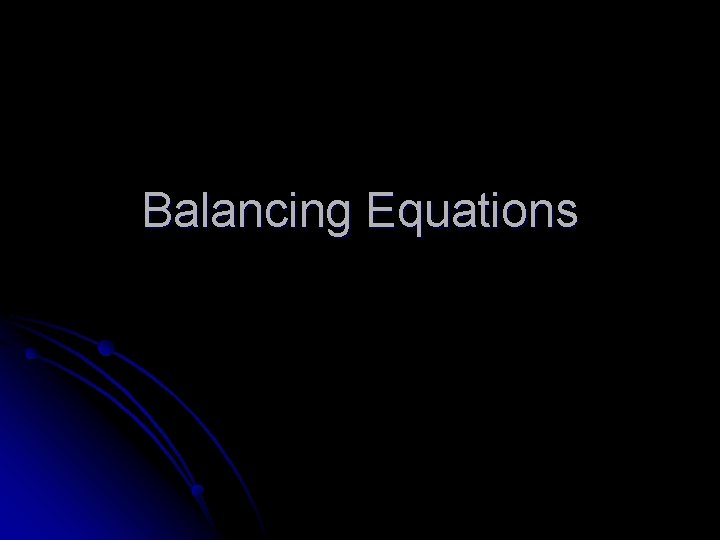Balancing Equations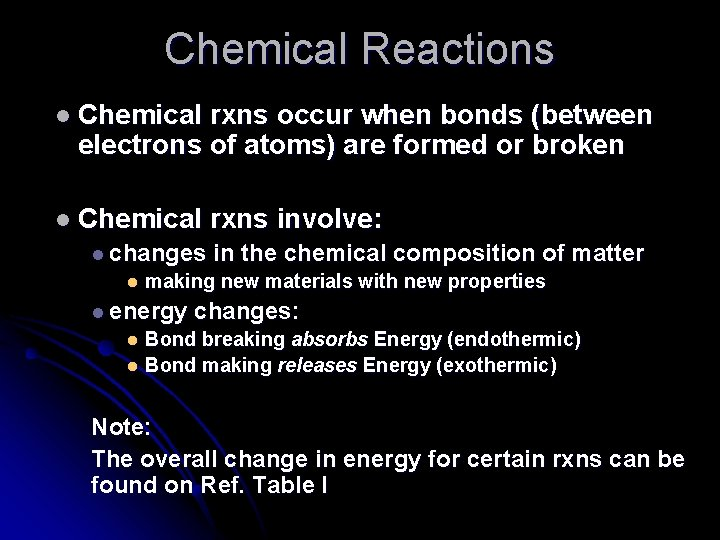Chemical Reactions l Chemical rxns occur when bonds (between electrons of atoms) are formed or broken l Chemical l changes l rxns involve: in the chemical composition of matter making new materials with new properties l energy changes: Bond breaking absorbs Energy (endothermic) l Bond making releases Energy (exothermic) l Note: The overall change in energy for certain rxns can be found on Ref. Table IChemical Equations Depict the kind of reactants and products and their relative amounts in a reaction. 4 Al (s) + 3 O 2 (g) → 2 Al 2 O 3 (s) The numbers in the front are called stoichiometric COEFFICIANTSSymbols Used in Equations l. Solid (s), Liquid (l), Gas (g) l. Aqueous solution (aq) (dissolved in water) l. Catalyst H 2 SO 4 l. Escaping gas ( ) or Pt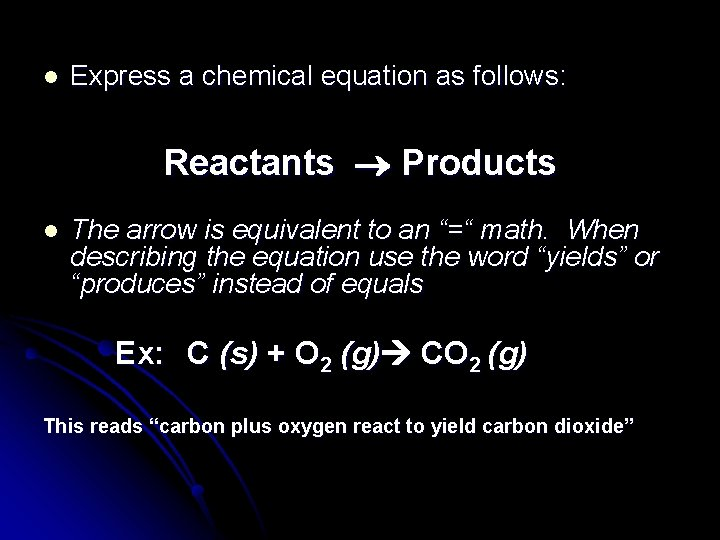l Express a chemical equation as follows: Reactants Products l The arrow is equivalent to an “=“ math. When describing the equation use the word “yields” or “produces” instead of equals Ex: C (s) + O 2 (g) CO 2 (g) This reads “carbon plus oxygen react to yield carbon dioxide”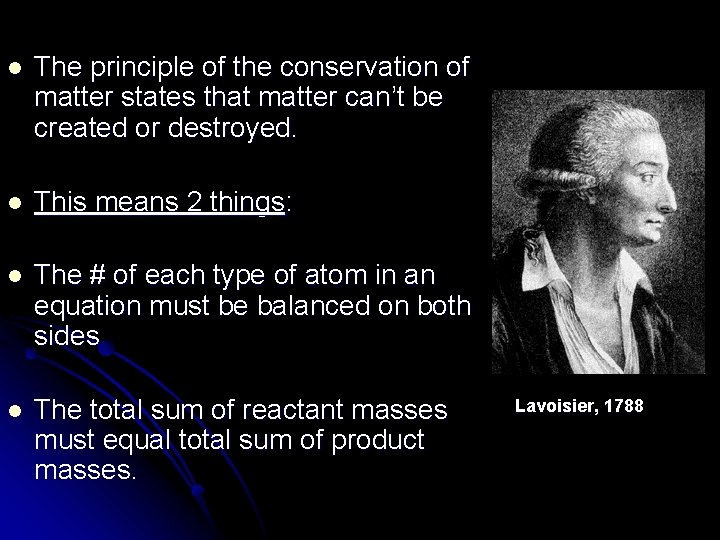l The principle of the conservation of matter states that matter can’t be created or destroyed. l This means 2 things: l The # of each type of atom in an equation must be balanced on both sides l The total sum of reactant masses must equal total sum of product masses. Lavoisier, 1788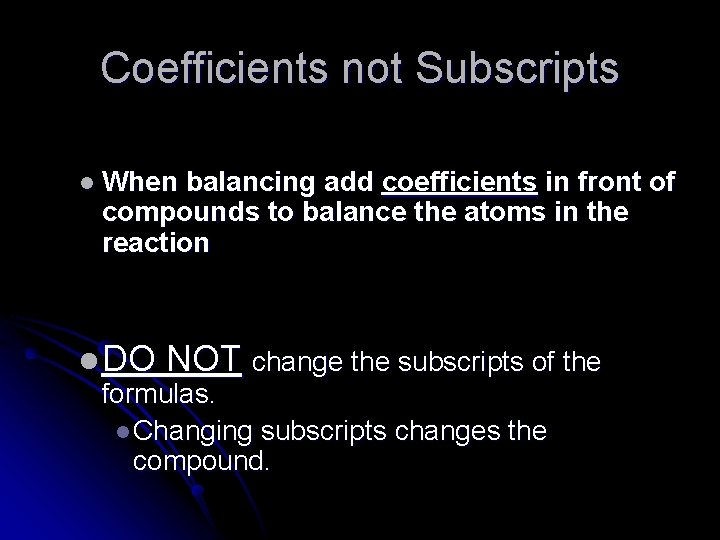Coefficients not Subscripts l When balancing add coefficients in front of compounds to balance the atoms in the reaction l DO NOT change the subscripts of the formulas. l Changing subscripts changes the compound.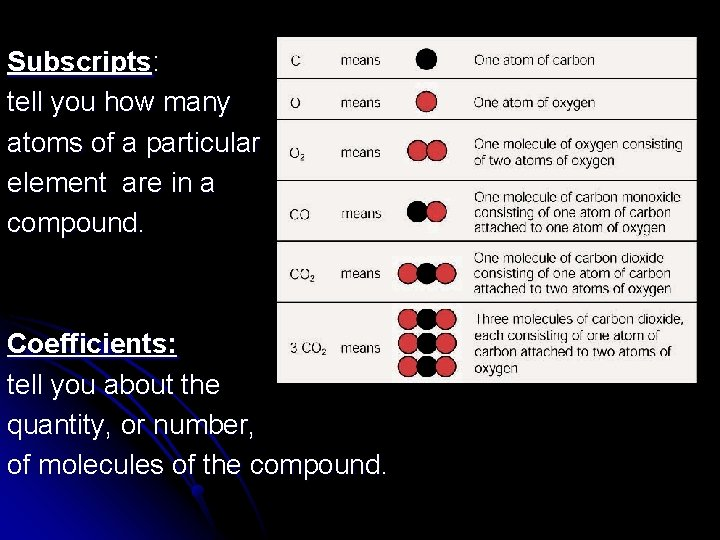Subscripts: tell you how many atoms of a particular element are in a compound. Coefficients: tell you about the quantity, or number, of molecules of the compound.Balancing a chemical equation: DO NOT CHANGE THE FORMULAS! l Find number of atoms for an element on left side. l Compare those against number of the atoms of the same element on right side. l Place coefficients in front of formulas so that left side has the same number of atoms as the right side for EACH element l Check your answer to see if: l The numbers of atoms on both sides of the equation balanced. l The coefficients are in the lowest possible whole number ratios.Helpful hints for balancing equations: l Take one element at a time, working left to right. l Save H for next to last, and O until last. l IF everything balances except for O, and there is no way to balance O with a whole number, double all the coefficients and try again. l Useful in combustion reactions! l (Shortcut) Polyatomic ions that appear on both sides of the equation should be balanced as independent units l Just circle them and think of them as one thing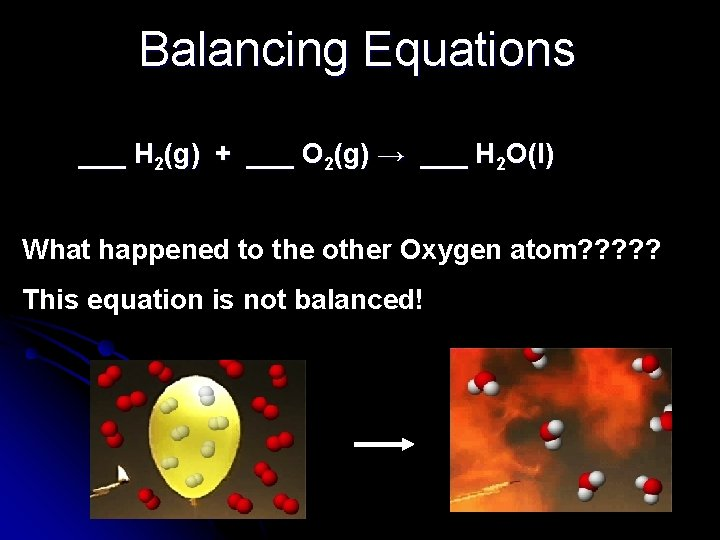Balancing Equations ___ H 2(g) + ___ O 2(g) → ___ H 2 O(l) What happened to the other Oxygen atom? ? ? This equation is not balanced!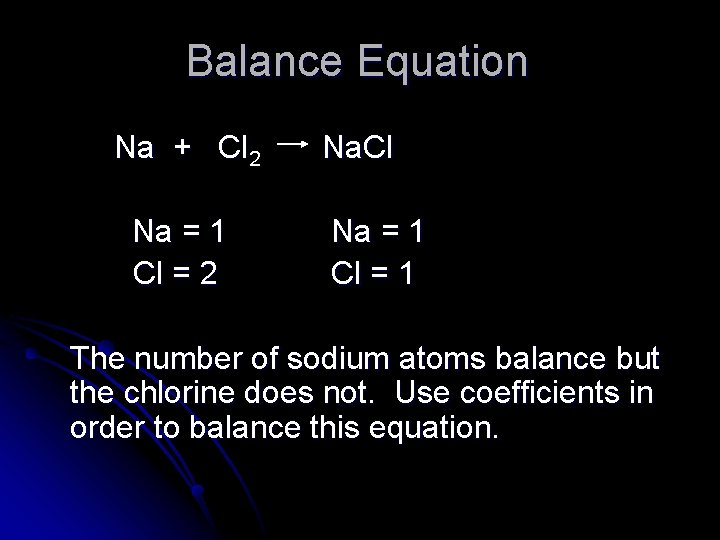Balance Equation Na + Cl 2 Na = 1 Cl = 2 Na. Cl Na = 1 Cl = 1 The number of sodium atoms balance but the chlorine does not. Use coefficients in order to balance this equation.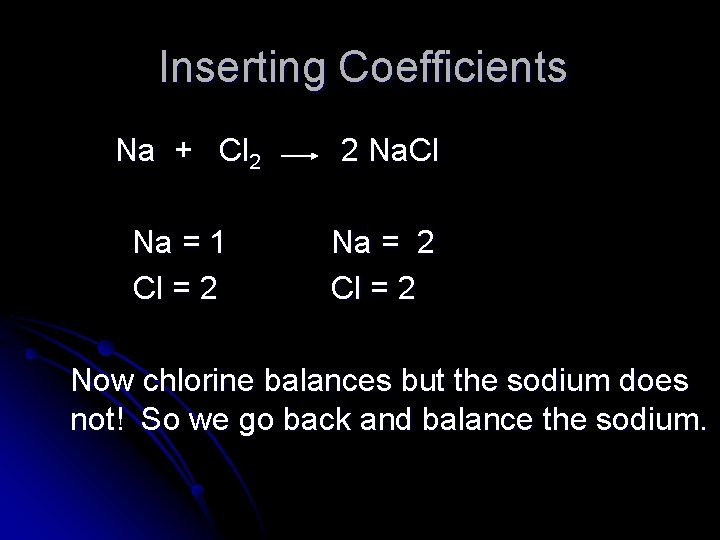Inserting Coefficients Na + Cl 2 2 Na. Cl Na = 1 Cl = 2 Na = 2 Cl = 2 Now chlorine balances but the sodium does not! So we go back and balance the sodium.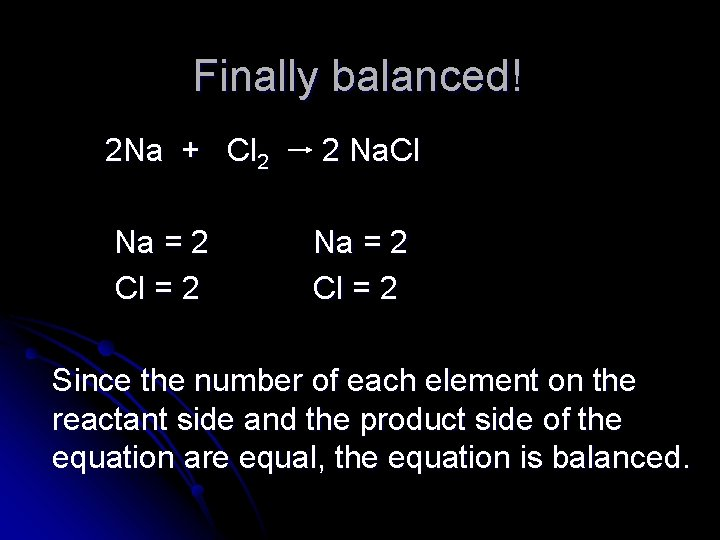Finally balanced! 2 Na + Cl 2 2 Na. Cl Na = 2 Cl = 2 Since the number of each element on the reactant side and the product side of the equation are equal, the equation is balanced.Balance Practice (Page 2 in Chemical Equations Packet) ___Al 2 O 3 + ___H 2 → ___H 2 O + ___Al ___Mg. I 2 + ___K → ___KI + ___Mg ___Al. F 3 → ___Al + ___F 2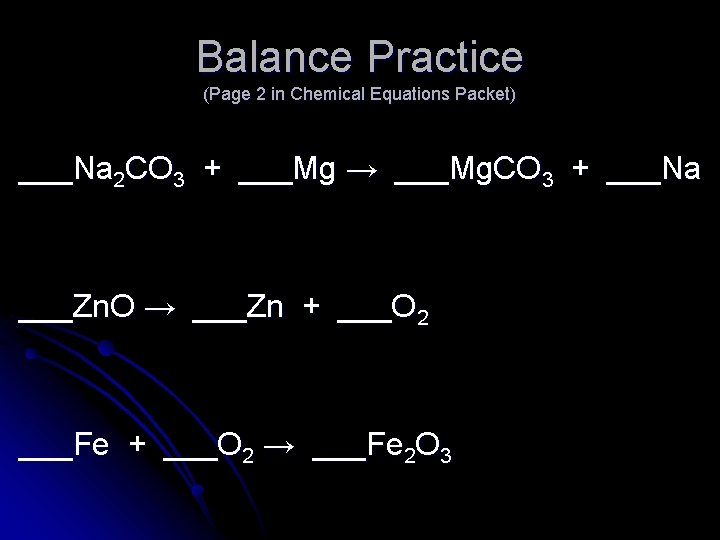Balance Practice (Page 2 in Chemical Equations Packet) ___Na 2 CO 3 + ___Mg → ___Mg. CO 3 + ___Na ___Zn. O → ___Zn + ___O 2 ___Fe + ___O 2 → ___Fe 2 O 3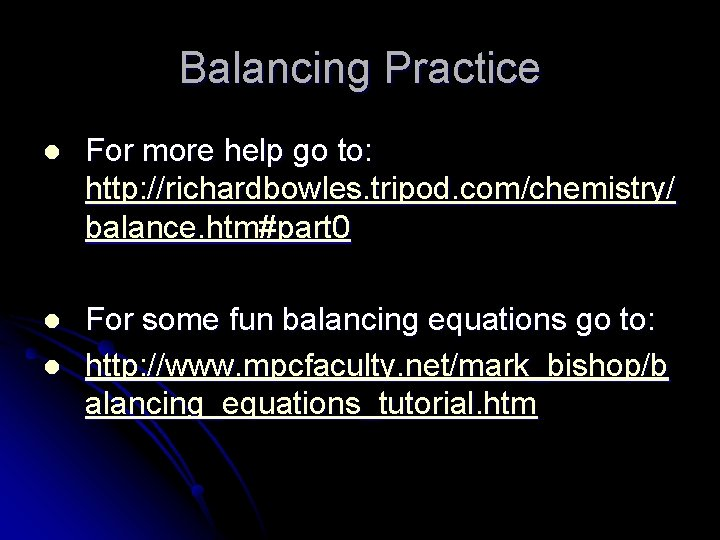Balancing Practice l For more help go to: http: //richardbowles. tripod. com/chemistry/ balance. htm#part 0 l For some fun balancing equations go to: http: //www. mpcfaculty. net/mark_bishop/b alancing_equations_tutorial. htm l